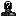## Jul. 23rd, 2011### 03:52 pm - НОД на Гоу

```/*
SYNOPSIS:

With GCC >= 4.6:
# gccgo gcd.go -o gcd-go
# ./gcd-go 11 22 33 44 121

With Google Go (6g for amd64, 8g for x86):
# 6g -o gcd-go.o  gcd.go
# 6l -o gcd-go-6g gcd-go.o
# ./gcd-go-6g 11 22 33 44 121

GCC makes dynamically linked binary,

*/

package main

// Both Google Go and GCC issue an error "imported and not used",
// if imported and not used :-)
import "fmt"
import "flag"
import "strconv"

func gcd2 (a, b uint) uint {
if b == 0 {
return a
}
/* 6g issues an error "function ends without a return statement",
if we use if ... {... return} else {... return}.
But GCC doesn't.
*/
return gcd2(b, a % b)
}

func gcdn (ns []uint) uint {
var r uint // zero by default
for i := range ns {
r = gcd2(r, ns[i])
}
return r
}

func main() {
flag.Parse() // without this 6g will give flag.NArg() = 0 next (WTF?)
n := flag.NArg()
if n > 0 {
ns := make([]uint, n) // We have garbage collector!

// Or: for i := range ns, since range of ns is equal to flag.NArg()
for i := 0; i < n; i++ {
// Drop the second return value (error code):
ns[i], _ = strconv.Atoui(flag.Arg(i))
}

g  := gcdn(ns)
fmt.Printf("%v\n", g)
}
}
```

https://github.com/ip1981/ebuilds/tree/master/dev-lang/golang(will be screened)